Function Repository Resource:

# GeoSpatialDistanceList

Get a list of spatial distances between consecutive pairs of locations

Contributed by: Peter Cullen Burbery
 ResourceFunction["GeoSpatialDistanceList"][{loc1,loc2,…}] returns the list of successive spatial distances between consecutive pairs of locations.

## Details

The spatial distance is the straight-line distance between two points on Earth, or the distance travelled if one could tunnel through the Earth from one point to the other.
The distances are returned in the unit system specified by \$UnitSystem.

## Examples

### Basic Examples (2)

Find the successive distances between your location, Null Island, which is the area of ocean near Africa with 0 latitude and 0 longitude, the North and South Poles, and the antipode of your location:

 In:=Out=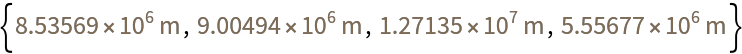Convert to kilometers:

 In:=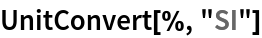Out=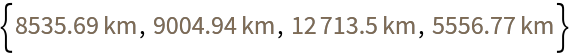### Applications (13)

A megacity, according to the United Nations Department of Economic and Social Affairs, is an urban agglomeration with 10 million inhabitants. Compute the positions for a list of megacities:

 In:=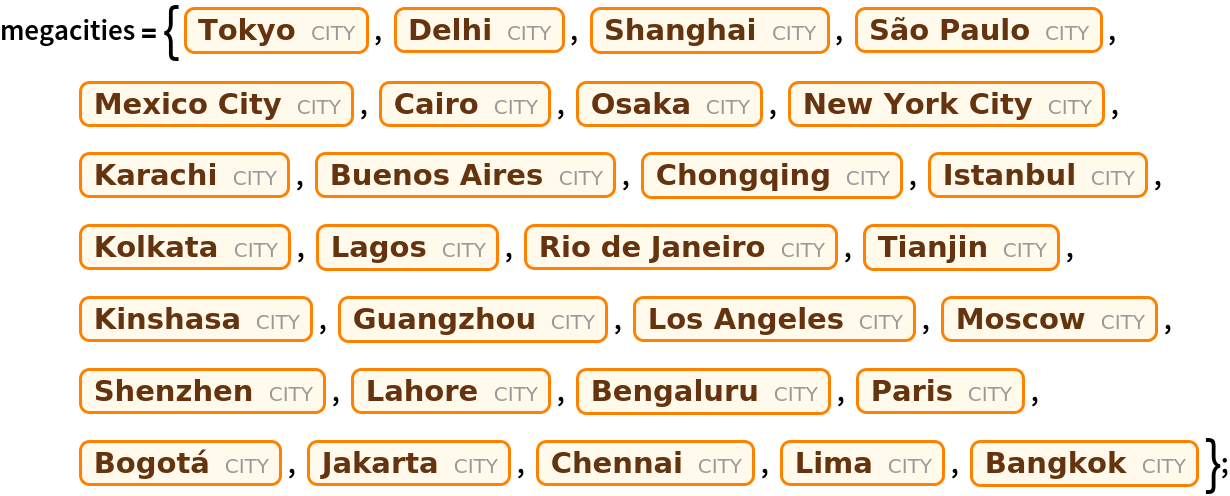In:=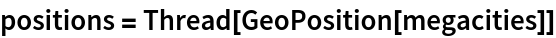Out=Find the successive spatial distances for a list of megacities:

 In:=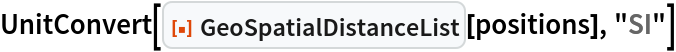Out=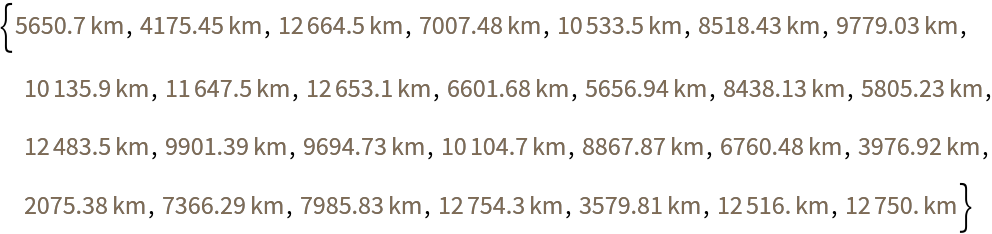Compare with the result of GeoDistanceList:

 In:=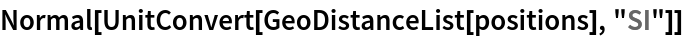Out=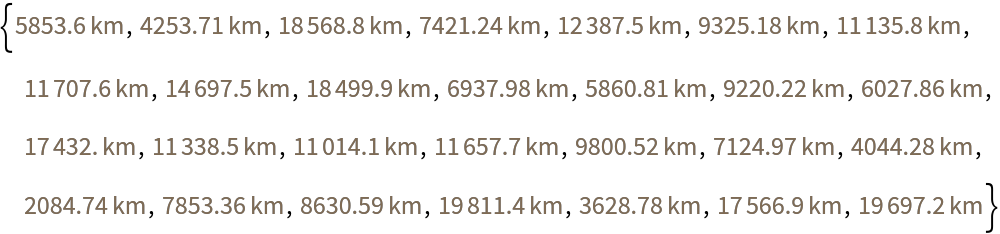Find the difference:

 In:=Out=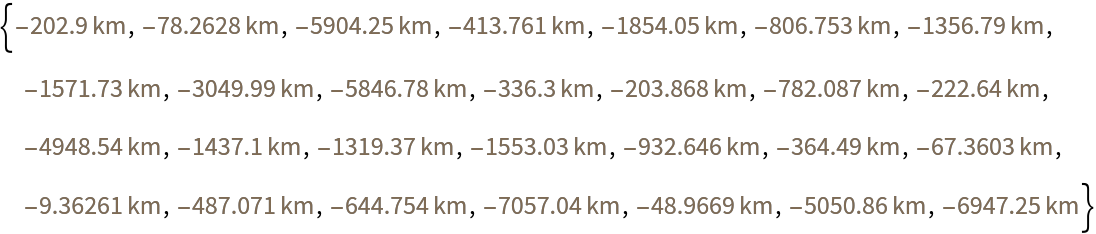Find the average decrease in distance if you could travel through the Earth:

 In:=Out=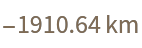Find how fast it would take to travel this distance at the speed of light:

 In:=Out=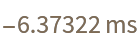Find the shortest path starting and ending at the two closest megacities to your location:

 In:=Out=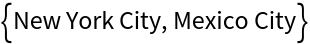Find the index of the cities:

 In:=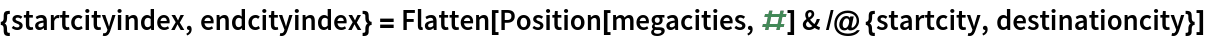Out=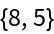Find the shortest tour of all mega-cities:

 In:=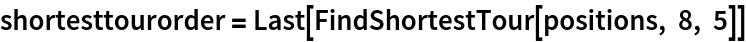Out=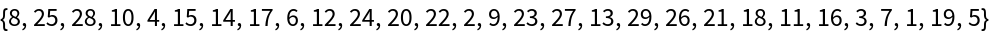Compare the distances with GeoDistance over Earth's surface and GeoSpatialDistance through space:

 In:=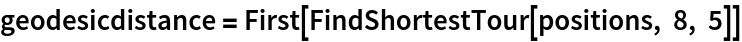Out=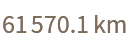In:=Out=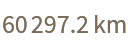Find the difference:

 In:=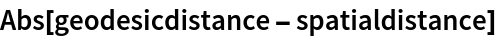Out=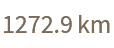Evaluate how fast this would take to travel at the speed of light:

 In:=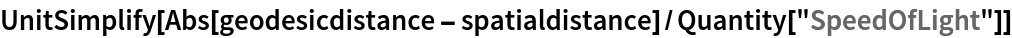Out=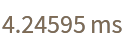Make a map of the cities with geo markers for each city:

 In:=Out=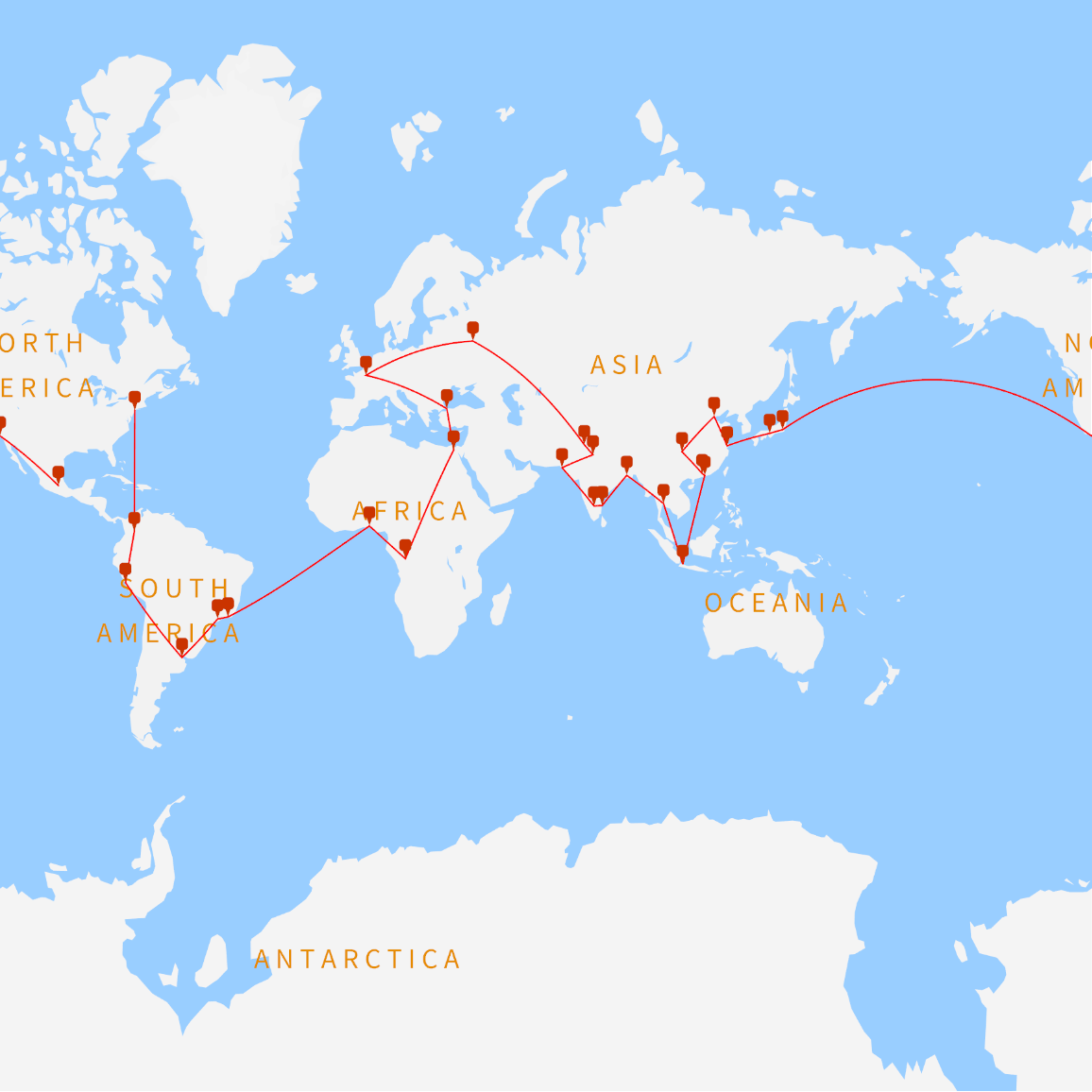Peter Burbery

## Version History

• 1.0.0 – 22 August 2022